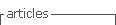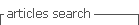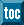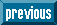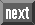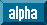## Indicators

•Cited by SciELO
•Access statistics

•Cited by Google
•Similars in SciELO
•Similars in Google

## Print version ISSN 0120-6230On-line version ISSN 2422-2844

#### Abstract

DIAZ DE LEON SANTIAGO, Juan et al. K-statistical operators for set mathematical morphology. Rev.fac.ing.univ. Antioquia [online]. 2009, n.48, pp.216-227. ISSN 0120-6230.

A generalization of the basic operators in mathematical morphology is presented in this paper. The resulting generalization lead to the construction of the k-statistical operators, due to its ability to obtain geometricaly the statistical descriptors within the range of the operator (Z={0,1}). These operators can be less strict as to the expected result as in the basic operators of dilation and erosion. Furthermore, a single k-statistical operator represents a functional complete set that includes erosion and dilation as particular cases. In a k-statistical operator, the condition to obtain the resulting set depends on whether the area (number of elements) of intersection between the translated structural set and the original set is at least equal to k. As a consecuence, a single parameter (k) is able to create a manifold of operators ranging from erosion to dilation. Hence, other kinds of openings and closings with new behavior are created as alternative solutions to binary image processing problems.

Keywords : Mathematical morphology; dilatation; erosion; opening; closing.

· abstract in Spanish     · text in Spanish     · Spanish (pdf )All the contents of this journal, except where otherwise noted, is licensed under a Creative Commons Attribution License